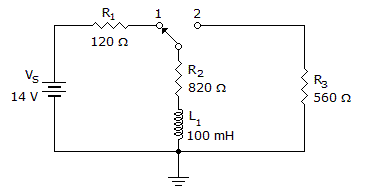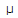# Electronics - Inductors - Discussion

### Discussion :: Inductors - General Questions (Q.No.2)

2.In the given circuit, what will the voltage be across R3 25s after the switch is moved to position 2?

 [A]. 2.88 V [B]. 5.9 V [C]. 8.34 V [D]. 14 V

Explanation:

No answer description available for this question.

 Nishant said: (Jan 14, 2011) Solution??

 Anvm said: (May 4, 2011) Please tell me the solution?

 Naseem Ul Aziz said: (May 8, 2011) Apply voltage divider that is when switch will be at position 2 v3=((r3)/(r3+r2))*vt=5.6 volt

 Ravi said: (Sep 15, 2011) Naseem is right.

 Isuru_R said: (Jan 3, 2012) When the switch is at "1" position I = 14/(120 + 820) I = 0.01489 Then we can find the current 25us after the switch is moved to position "2" using the equation I2 = I * e^(-tR/L) Then... from(R3* I2 ) we get the voltage across the resistor "R3". Substitute I = 0.01489 A ; t = 25x10^(-6) s ; R = (820 + 560) ; and L = 0.1 H Then you will get (I2*R3) = 5.9 V , which gaves us the required solution....!

 Sambhaji Gawande said: (Jan 30, 2012) Correct solution by Isuru_R.

 Latha said: (Mar 18, 2012) Naseem then how will you consider the given time?

 Preetha said: (Aug 24, 2012) @Isuru is correct.

 Clint said: (Aug 13, 2013) @Isuru, @Sambhaji, @Preetha, could you please solve I2 ? I didn't get the correct answer.

 Arun said: (Oct 25, 2013) @ clint Use the formula I2= I * (1- e^-t(R/L))

 Mayank said: (Oct 28, 2016) Did we ignore inductance when the switch is at position one due to its very less magnitude?

 Syed Shams Ul Arifeen Shah said: (Dec 13, 2016) @Mayank, Yes we ignore the inductance because in DC source, frequency is zero so that inductor behave like short wire (XL = 2 * pi * F * L) here F = 0, XL = 0.

 Chandhu said: (Jan 2, 2017) Open switch are there r1 then why voltage flow r3?

 Chandhu said: (Jan 2, 2017) Open switch are there r1 then why voltage flow r3?

 Ashfaque said: (Apr 21, 2018) @All. An Inductor is a short circuit to dc. Then why we would be bothered about 25 microseconds. I think you are correct @Naseem.

 Priyanka said: (Jul 1, 2019) @Clint. I2 = I*(e^(t(R/L)). Then I2 = 10.54*10^-3. Therefore voltage across R3 = I2*R3 =5.905 v.

 Oliver said: (Dec 23, 2019) Yes, but to be clear, inductors are shorted (VL = 0) at DC only when STEADY STATE is reached (around 5 time constants). Though the question did not mention it, it was assumed to be that way which is why its more confusing.